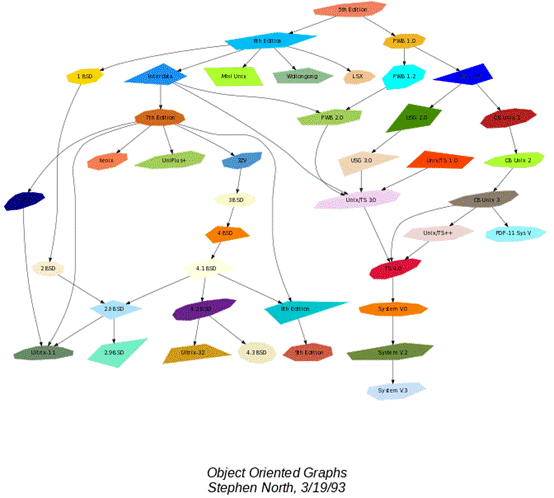2015年11月10日更新 在实践中，我又发现了一些`graphviz`的有趣的特性，比如`时序图``rank`以及`图片节点`等。在这里一并更新。

##前言

###graphviz简介

`graphviz`中包含了众多的布局器：

• `dot` 默认布局方式，主要用于有向图
• `neato` 基于spring-model(又称force-based)算法
• `twopi` 径向布局
• `circo` 圆环布局
• `fdp` 用于无向图

`graphviz`的设计初衷是对`有向图/无向图`等进行自动布局，开发人员使用dot脚本定义图形元素，然后选择算法进行布局，最终导出结果。

• 定义一个图，并向图中添加需要的顶点和边
• 为顶点和边添加样式
• 使用布局引擎进行绘制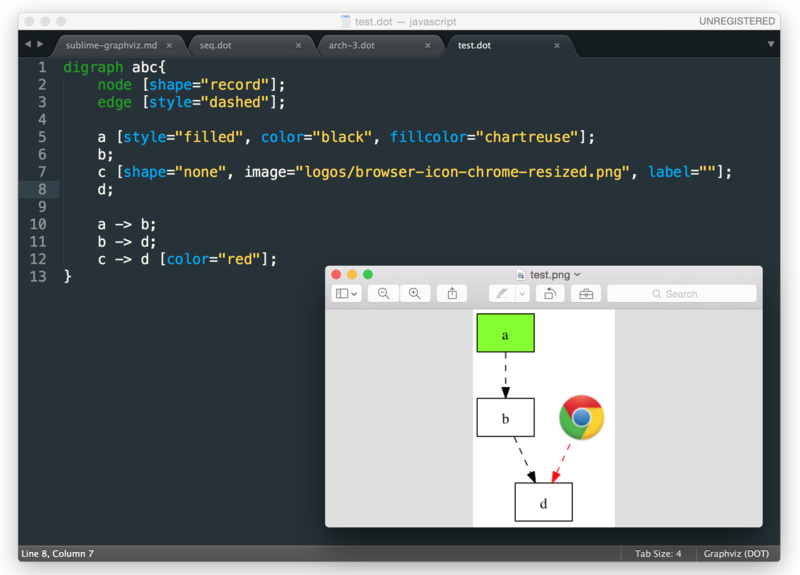###基础知识

graphviz包含3中元素，`图``顶点``边`。每个元素都可以具有各自的属性，用来定义字体，样式，颜色，形状等。下面是一些简单的示例，可以帮助我们快速的了解graphviz的基本用法。

####第一个graphviz图

``````digraph abc{
a;
b;
c;
d;

a -> b;
b -> d;
c -> d;
}
``````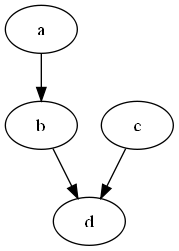####定义顶点和边的样式

`digraph`的花括号内，添加顶点和边的新定义：

``````node [shape="record"];
edge [style="dashed"];
``````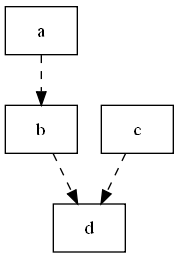####进一步修改顶点和边样式

``````digraph abc{
node [shape="record"];
edge [style="dashed"];

a [style="filled", color="black", fillcolor="chartreuse"];
b;
c;
d;

a -> b;
b -> d;
c -> d [color="red"];
}
``````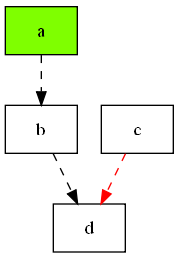####以图片为节点

``````digraph abc{
node [shape="record"];
edge [style="dashed"];

a [style="filled", color="black", fillcolor="chartreuse"];
b;
c [shape="none", image="logos/browser-icon-chrome-resized.png", label=""];
d;

a -> b;
b -> d;
c -> d [color="red"];
}
``````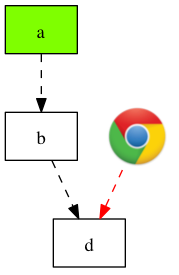###子图的绘制

graphviz支持子图，即图中的部分节点和边相对对立(软件的模块划分经常如此)。比如，我们可以将顶点c和d归为一个子图：

``````digraph abc{

node [shape="record"];
edge [style="dashed"];

a [style="filled", color="black", fillcolor="chartreuse"];
b;

subgraph cluster_cd{
label="c and d";
bgcolor="mintcream";
c;
d;
}

a -> b;
b -> d;
c -> d [color="red"];
}
``````

`c``d`划分到`cluster_cd`这个子图中，标签为`c and d`,并添加背景色，以方便与主图区分开，绘制结果如下：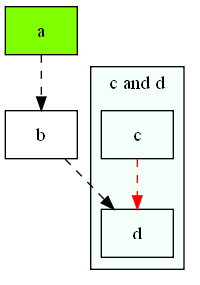####数据结构的可视化

#####一个hash表的数据结构

``````struct st_hash_type {
int (*compare) ();
int (*hash) ();
};

struct st_table_entry {
unsigned int hash;
char *key;
char *record;
st_table_entry *next;
};

struct st_table {
struct st_hash_type *type;
int num_bins; /* slot count */
int num_entries; /* total number of entries */
struct st_table_entry **bins; /* slot */
};
``````

#####绘制hash表的数据结构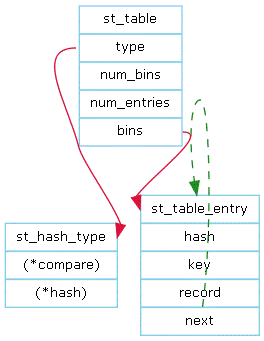``````digraph st2{
fontname = "Verdana";
fontsize = 10;
rankdir=TB;

node [fontname = "Verdana", fontsize = 10, color="skyblue", shape="record"];

edge [fontname = "Verdana", fontsize = 10, color="crimson", style="solid"];

st_table [label="{st_table|<type>type|num_bins|num_entries|<bins>bins}"];

}
``````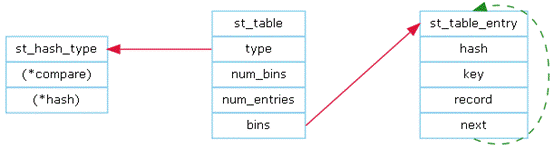####hash表的实例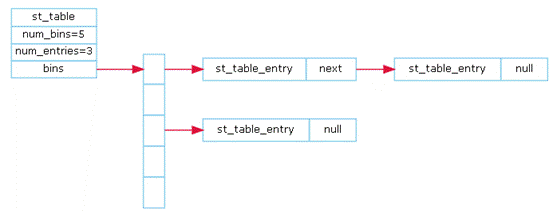``````digraph st{
fontname = "Verdana";
fontsize = 10;
rankdir = LR;
rotate = 90;

node [ shape="record", width=.1, height=.1];
node [fontname = "Verdana", fontsize = 10, color="skyblue", shape="record"];

edge [fontname = "Verdana", fontsize = 10, color="crimson", style="solid"];
node [shape="plaintext"];

st_table [label=<
<table border="0" cellborder="1" cellspacing="0" align="left">
<tr>
<td>st_table</td>
</tr>
<tr>
<td>num_bins=5</td>
</tr>
<tr>
<td>num_entries=3</td>
</tr>
<tr>
<td port="bins">bins</td>
</tr>
</table>
>];

node [shape="record"];
num_bins [label=" <b1> | <b2> | <b3> | <b4> | <b5> ", height=2];
node[ width=2 ];

entry_1 [label="{<e>st_table_entry|<next>next}"];
entry_2 [label="{<e>st_table_entry|<next>null}"];
entry_3 [label="{<e>st_table_entry|<next>null}"];

st_table:bins -> num_bins:b1;
num_bins:b1 -> entry_1:e;
entry_1:next -> entry_2:e;
num_bins:b3 -> entry_3:e;
}
``````

####软件模块组成图

Apache httpd 模块关系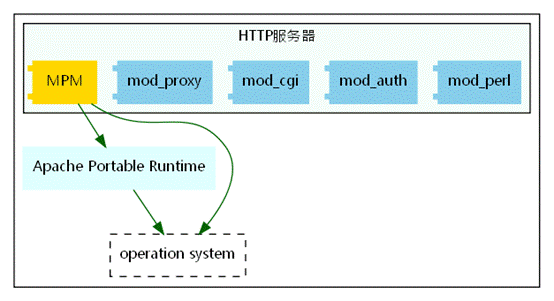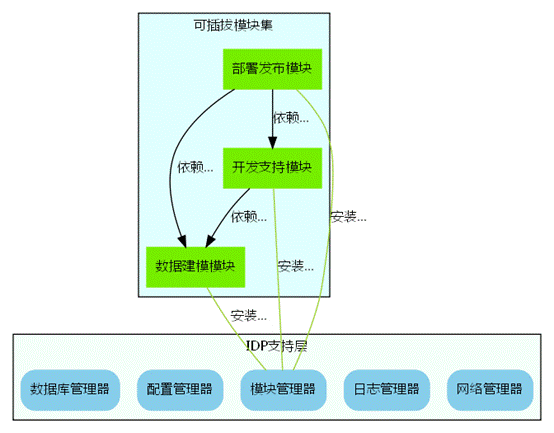IDP支持层为一个相对独立的子系统，其中包括如数据库管理器，配置信息管理器等模块，另外为了提供更大的灵活性，将很多其他的模块抽取出来作为外部模块，而支持层提供一个模块管理器，来负责加载/卸载这些外部的模块集合。

``````digraph idp_modules{

rankdir = TB;
fontname = "Microsoft YaHei";
fontsize = 12;

node [ fontname = "Microsoft YaHei", fontsize = 12, shape = "record" ];
edge [ fontname = "Microsoft YaHei", fontsize = 12 ];

subgraph cluster_sl{
label="IDP支持层";
bgcolor="mintcream";
node [shape="Mrecord", color="skyblue", style="filled"];
network_mgr [label="网络管理器"];
log_mgr [label="日志管理器"];
module_mgr [label="模块管理器"];
conf_mgr [label="配置管理器"];
db_mgr [label="数据库管理器"];
};

subgraph cluster_md{
label="可插拔模块集";
bgcolor="lightcyan";
node [color="chartreuse2", style="filled"];
mod_dev [label="开发支持模块"];
mod_dm [label="数据建模模块"];
mod_dp [label="部署发布模块"];
};

mod_dp -> mod_dev [label="依赖..."];
mod_dp -> mod_dm [label="依赖..."];
mod_dp -> module_mgr [label="安装...", color="yellowgreen", arrowhead="none"];
mod_dev -> mod_dm [label="依赖..."];
mod_dev -> module_mgr [label="安装...", color="yellowgreen", arrowhead="none"];
mod_dm -> module_mgr [label="安装...", color="yellowgreen", arrowhead="none"];
}
``````

####状态图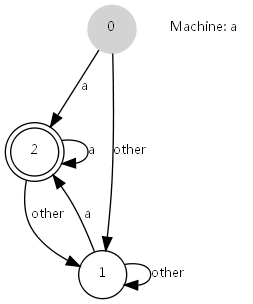``````digraph automata_0 {
size = "8.5, 11";
fontname = "Microsoft YaHei";
fontsize = 10;

node [shape = circle, fontname = "Microsoft YaHei", fontsize = 10];
edge [fontname = "Microsoft YaHei", fontsize = 10];

0 [ style = filled, color=lightgrey ];
2 [ shape = doublecircle ];

0 -> 2 [ label = "a " ];
0 -> 1 [ label = "other " ];
1 -> 2 [ label = "a " ];
1 -> 1 [ label = "other " ];
2 -> 2 [ label = "a " ];
2 -> 1 [ label = "other " ];

"Machine: a" [ shape = plaintext ];
}
``````

####OSGi中模块的生命周期图

OSGi中，模块具有生命周期，从安装到卸载，可能的状态具有已安装，已就绪，正在启动，已启动，正在停止，已卸载等。如下图所示：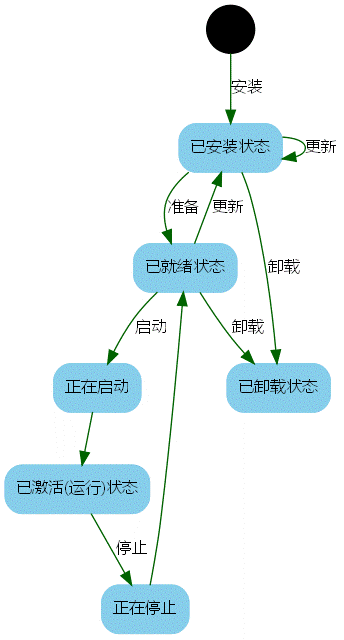``````digraph module_lc{
rankdir=TB;
fontname = "Microsoft YaHei";
fontsize = 12;

node [fontname = "Microsoft YaHei", fontsize = 12, shape = "Mrecord", color="skyblue", style="filled"];
edge [fontname = "Microsoft YaHei", fontsize = 12, color="darkgreen" ];

installed [label="已安装状态"];
resolved [label="已就绪状态"];
uninstalled [label="已卸载状态"];
starting [label="正在启动"];
active [label="已激活(运行)状态"];
stopping [label="正在停止"];
start [label="", shape="circle", width=0.5, fixedsize=true, style="filled", color="black"];

start -> installed [label="安装"];
installed -> uninstalled [label="卸载"];
installed -> resolved [label="准备"];
installed -> installed [label="更新"];
resolved -> installed [label="更新"];
resolved -> uninstalled [label="卸载"];
resolved -> starting [label="启动"];
starting -> active [label=""];
active -> stopping [label="停止"];
stopping -> resolved [label=""];
}
``````

###其他实例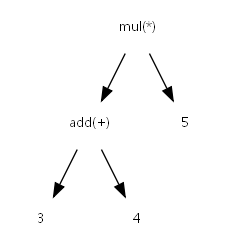``````digraph ast{
fontname = "Microsoft YaHei";
fontsize = 10;

node [shape = circle, fontname = "Microsoft YaHei", fontsize = 10];
edge [fontname = "Microsoft YaHei", fontsize = 10];
node [shape="plaintext"];

mul [label="mul(*)"];

mul -> 5;
}
``````

####简单的UML类图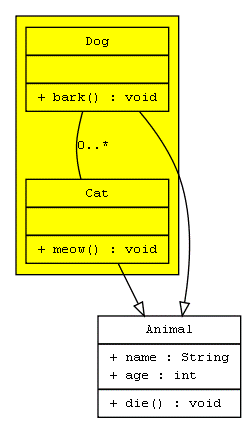``````digraph G{

fontname = "Courier New"
fontsize = 10

node [ fontname = "Courier New", fontsize = 10, shape = "record" ];
edge [ fontname = "Courier New", fontsize = 10 ];

Animal [ label = "{Animal |+ name : String\l+ age : int\l|+ die() : void\l}" ];

subgraph clusterAnimalImpl{
bgcolor="yellow"
Dog [ label = "{Dog||+ bark() : void\l}" ];
Cat [ label = "{Cat||+ meow() : void\l}" ];
};

edge [ arrowhead = "empty" ];

Dog->Animal;
Cat->Animal;
}
``````

####状态图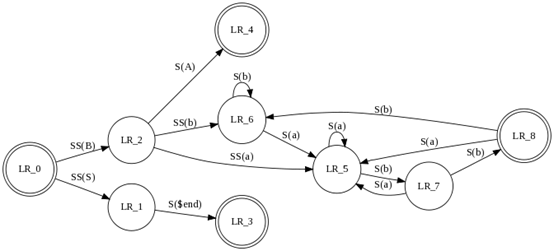``````digraph finite_state_machine {
rankdir = LR;
size = "8,5"

node [shape = doublecircle];

LR_0 LR_3 LR_4 LR_8;

node [shape = circle];

LR_0 -> LR_2 [ label = "SS(B)" ];
LR_0 -> LR_1 [ label = "SS(S)" ];
LR_1 -> LR_3 [ label = "S(\$end)" ];
LR_2 -> LR_6 [ label = "SS(b)" ];
LR_2 -> LR_5 [ label = "SS(a)" ];
LR_2 -> LR_4 [ label = "S(A)" ];
LR_5 -> LR_7 [ label = "S(b)" ];
LR_5 -> LR_5 [ label = "S(a)" ];
LR_6 -> LR_6 [ label = "S(b)" ];
LR_6 -> LR_5 [ label = "S(a)" ];
LR_7 -> LR_8 [ label = "S(b)" ];
LR_7 -> LR_5 [ label = "S(a)" ];
LR_8 -> LR_6 [ label = "S(b)" ];
LR_8 -> LR_5 [ label = "S(a)" ];
}
``````

#### 时序图¶

``````digraph G {
rankdir="LR";
node[shape="point", width=0, height=0];

{
rank="same";
edge[style="solided"];
LC[shape="plaintext"];
LC -> step00 -> step01 -> step02 -> step03 -> step04 -> step05;
}

{
rank="same";
edge[style="solided"];
Agency[shape="plaintext"];
Agency -> step10 -> step11 -> step12 -> step13 -> step14 -> step15;
}

{
rank="same";
edge[style="solided"];
Agent[shape="plaintext"];
Agent -> step20 -> step21 -> step22 -> step23 -> step24 -> step25;
}

step00 -> step10 [label="sends email new custumer", arrowhead="normal"];
step13 -> step23 [label="forward to", arrowhead="normal"];
step24 -> step14;
}
``````

`rankdir="LR"`表示，布局从左`L`到右`R`。可以看到，在代码中有`{}`括起来的部分。

``````{
rank="same";
edge[style="solided"];
Agency[shape="plaintext"];
Agency -> step10 -> step11 -> step12 -> step13 -> step14 -> step15;
}
``````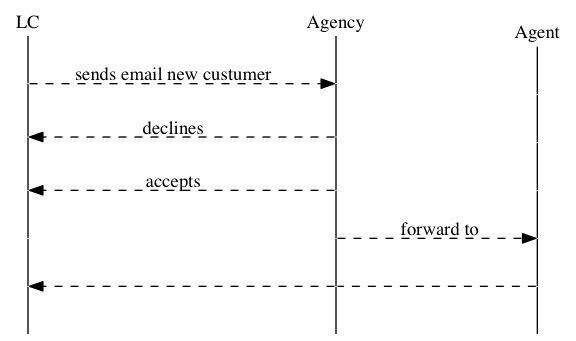###附录